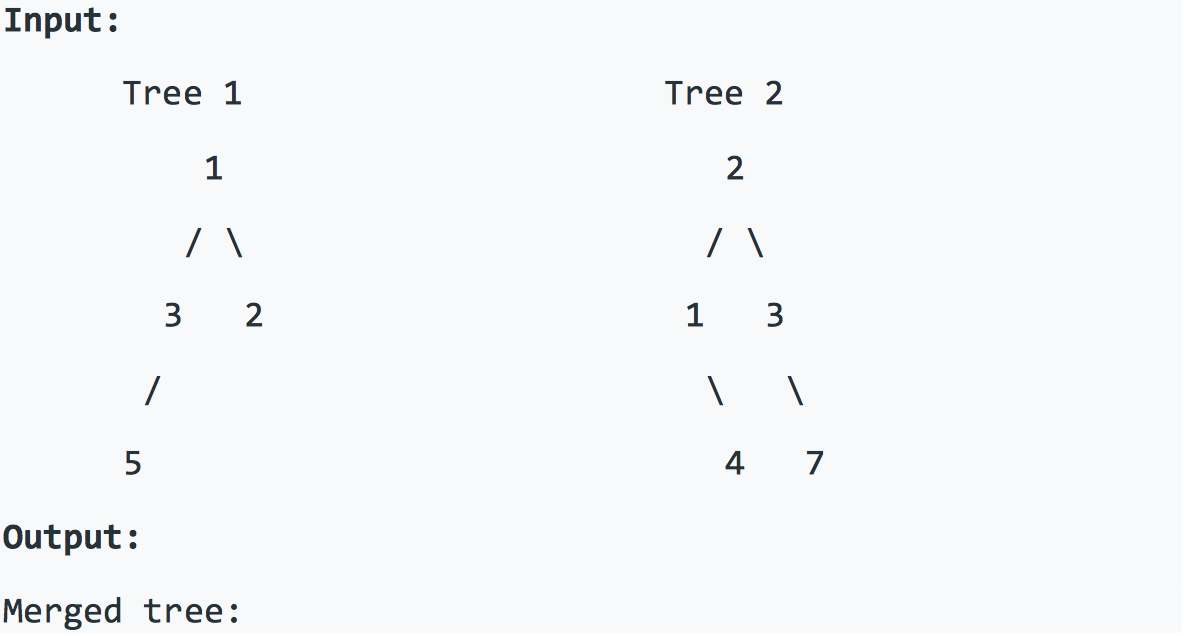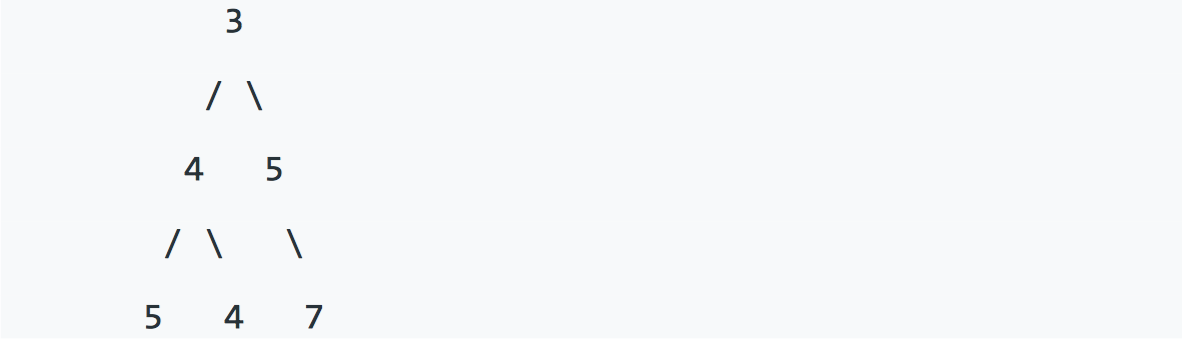You need to merge them into a new binary tree. The merge rule is that if two nodes overlap, then sum node values up as the new value of the merged node. Otherwise, the NOT null node will be used as the node of new tree.``````#include <iostream>

using namespace std;
class Node{
public:
int value;
Node * left;
Node * right;

public:
Node(int value);
~Node();
};

class Solution {
public:
Node * merge(Node * t1, Node * t2) {
if (!t1) return t2;
if (!t2) return t1;
Node * res = new Node(t1->value + t2->value);
res->left = merge(t1->left, t2->left);
res->right = merge(t1->right, t2->right);
return res;
}
};
int main(int argc, char *argv[]) {

}
``````